# Punjab Board (PSEB) Question Paper for Class 10th Maths 2016 In PDF

## PSEB Matriculation (10th Standard) Maths Exam Question Papers 2016 with Solutions – Free Download

PSEB (Punjab board) Public Exam Class 10 Maths 2016 Question Paper (A) with solutions are provided by the subject experts at BYJU’S. These solutions have detailed explanations along with the images which will help the students in understanding concepts involved in the questions. Also, the maths previous years question papers of Punjab board annual exam are available at BYJU’S, which can be downloaded easily in a pdf form to help the students in their exam preparation. The solutions for all these question papers of PSEB Class 10 Maths are also given so that students can verify them after solving by themselves. Students can solve these Punjab Board (PSEB) Question Paper for Class 10th Maths 2016 In PDF with Solutions and get used to answering a wide range of questions.

### PSEB Class 10 Mathematics Question Paper 2016 Paper A with Solutions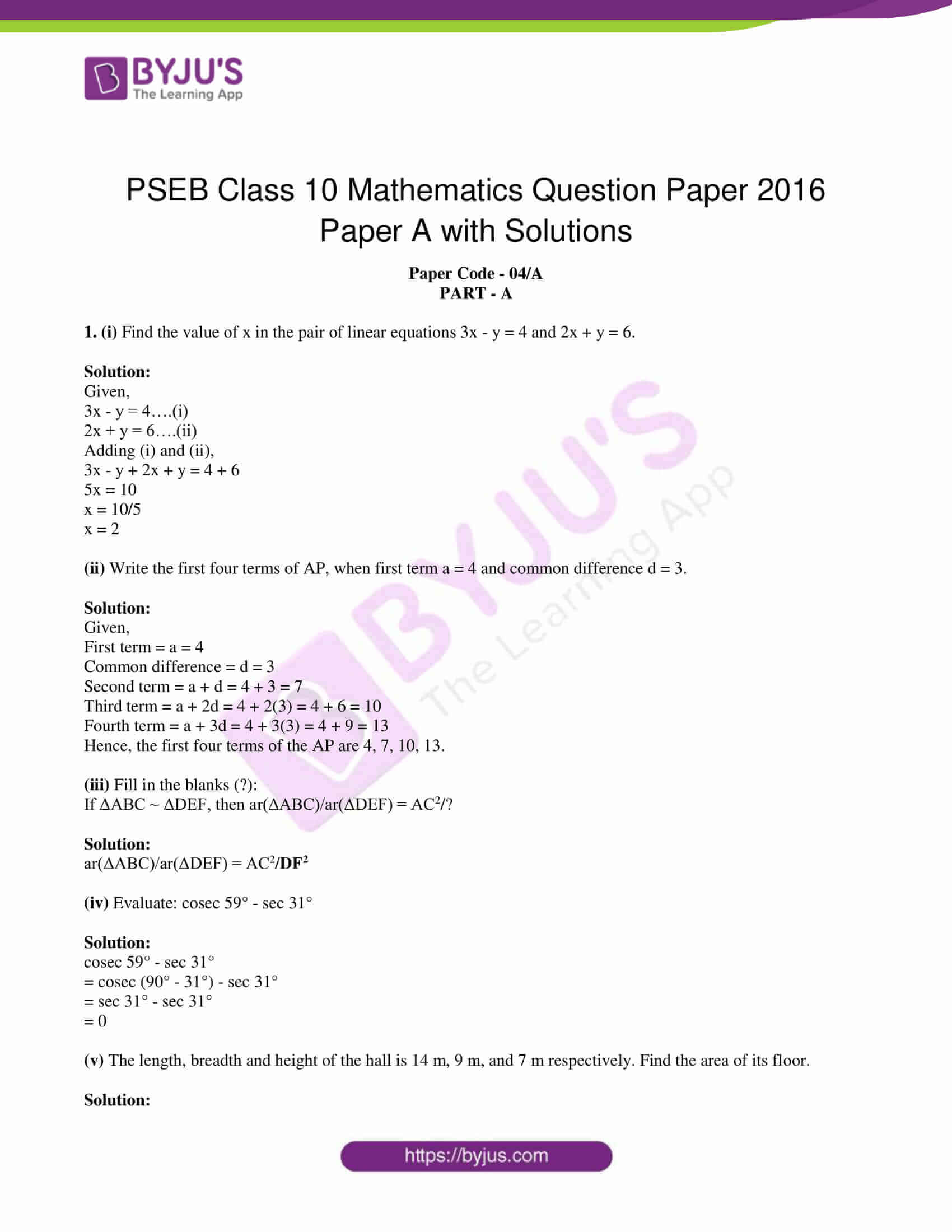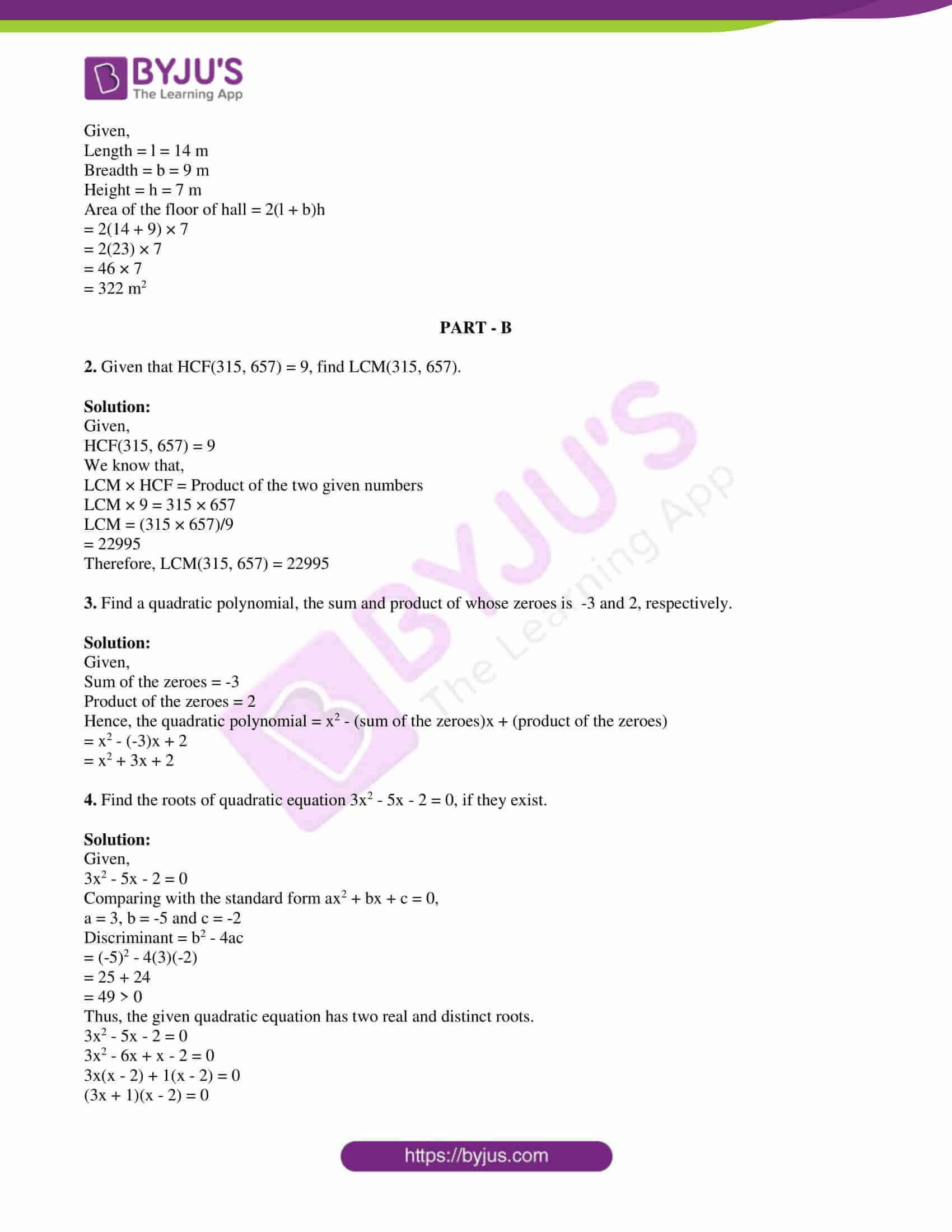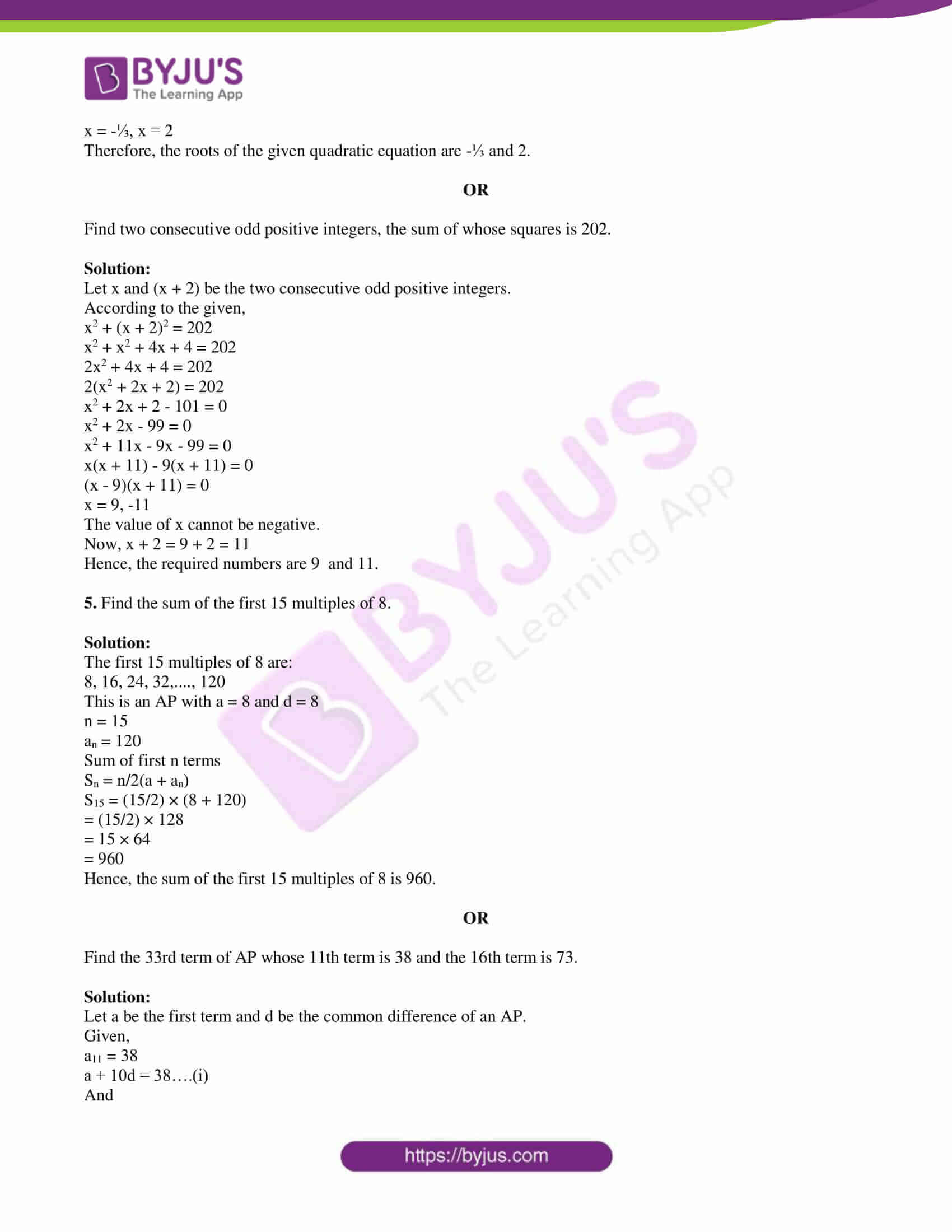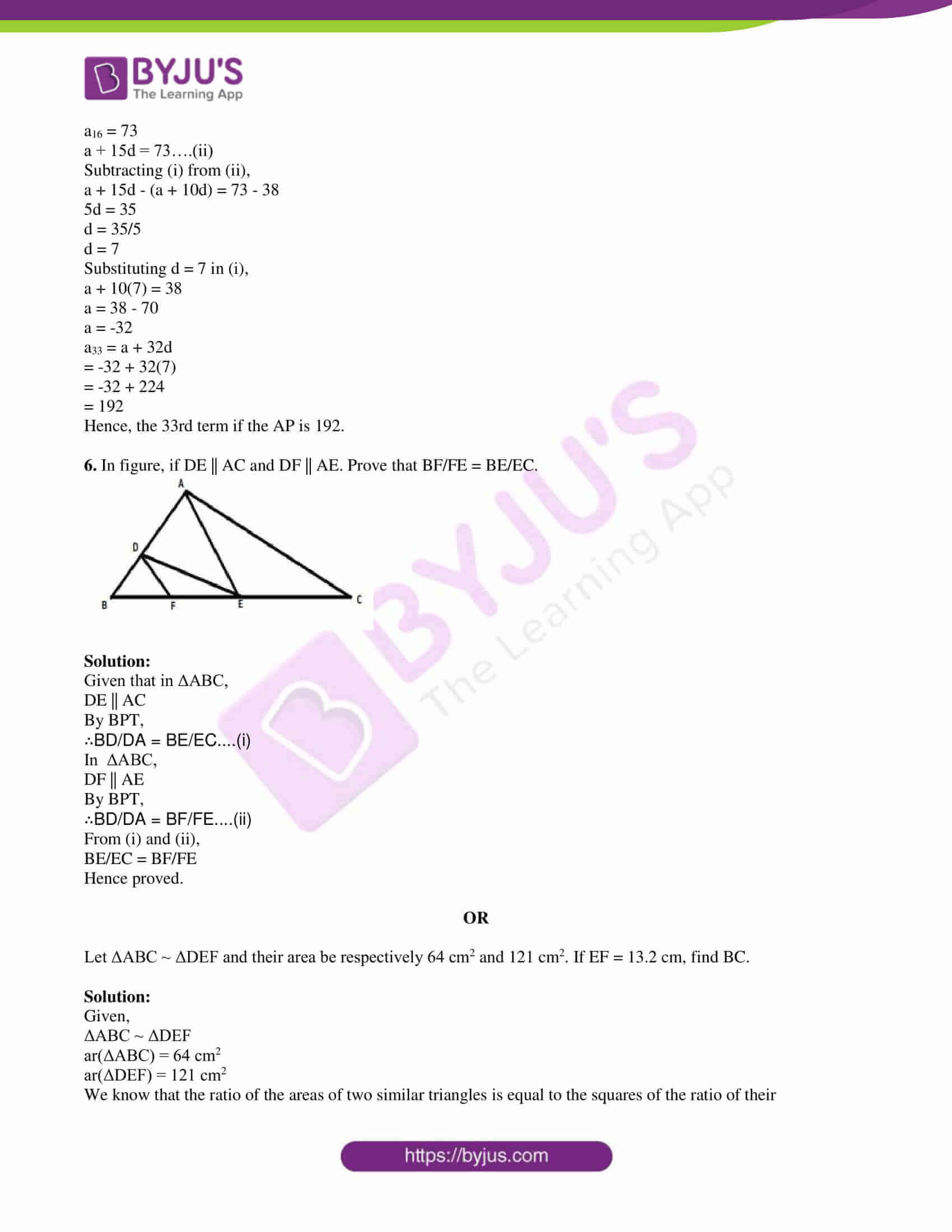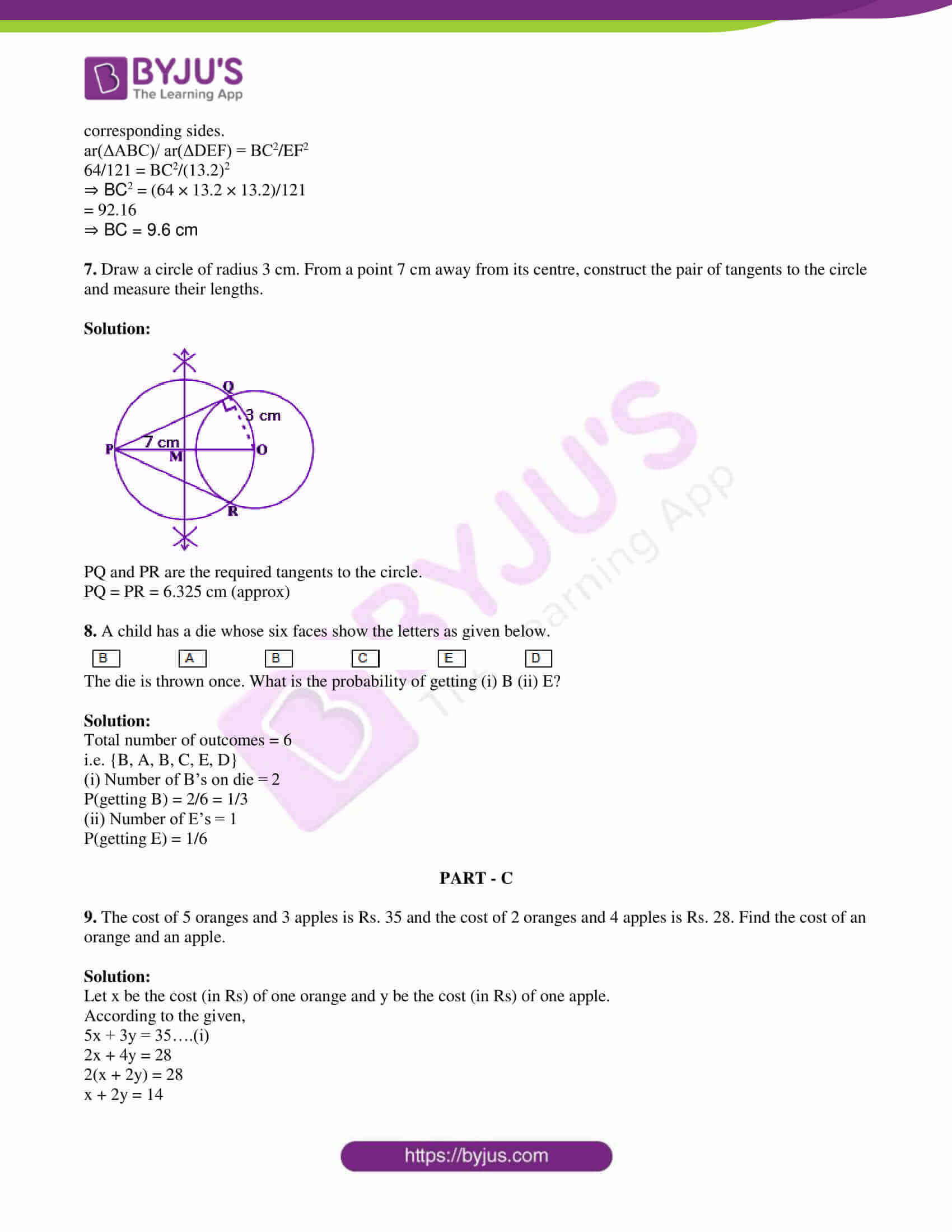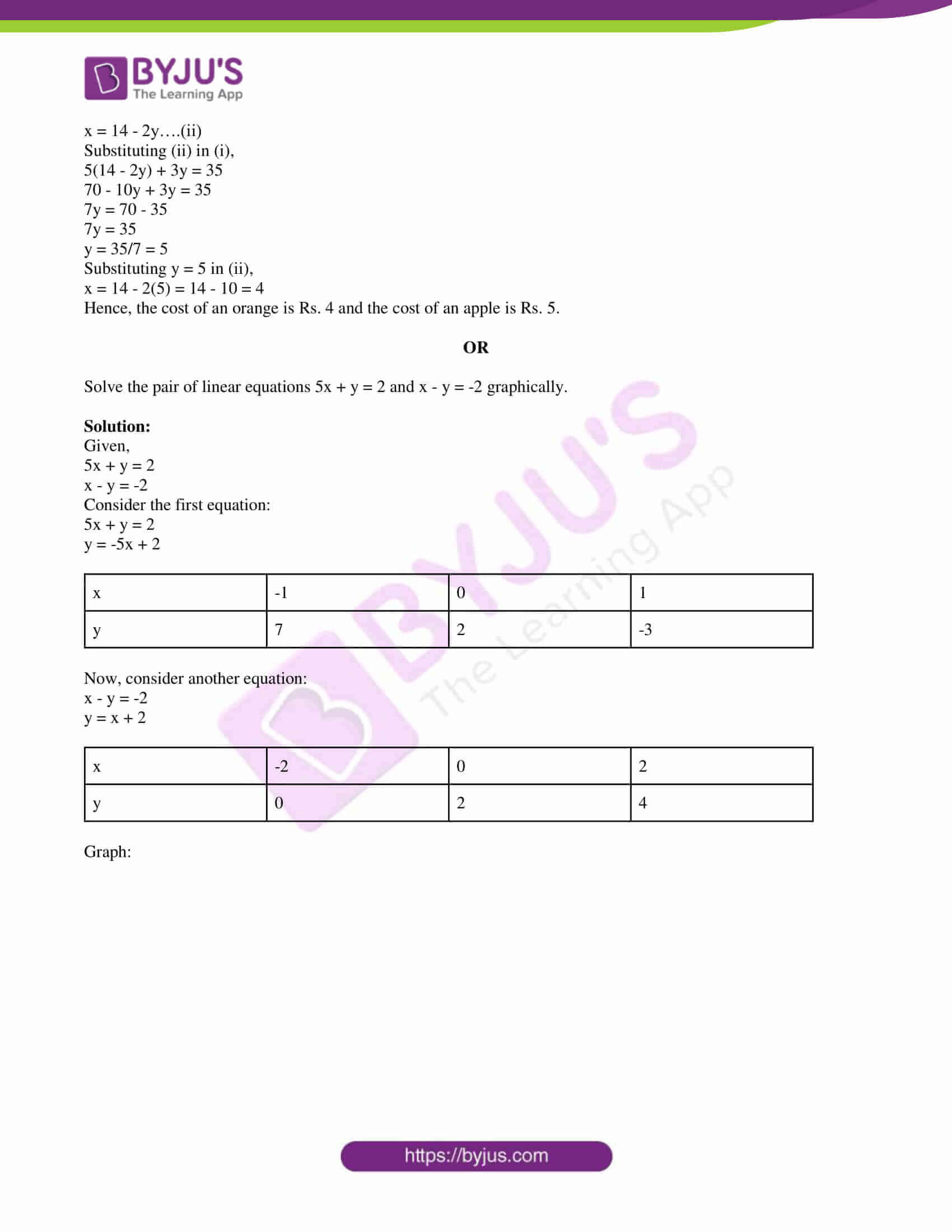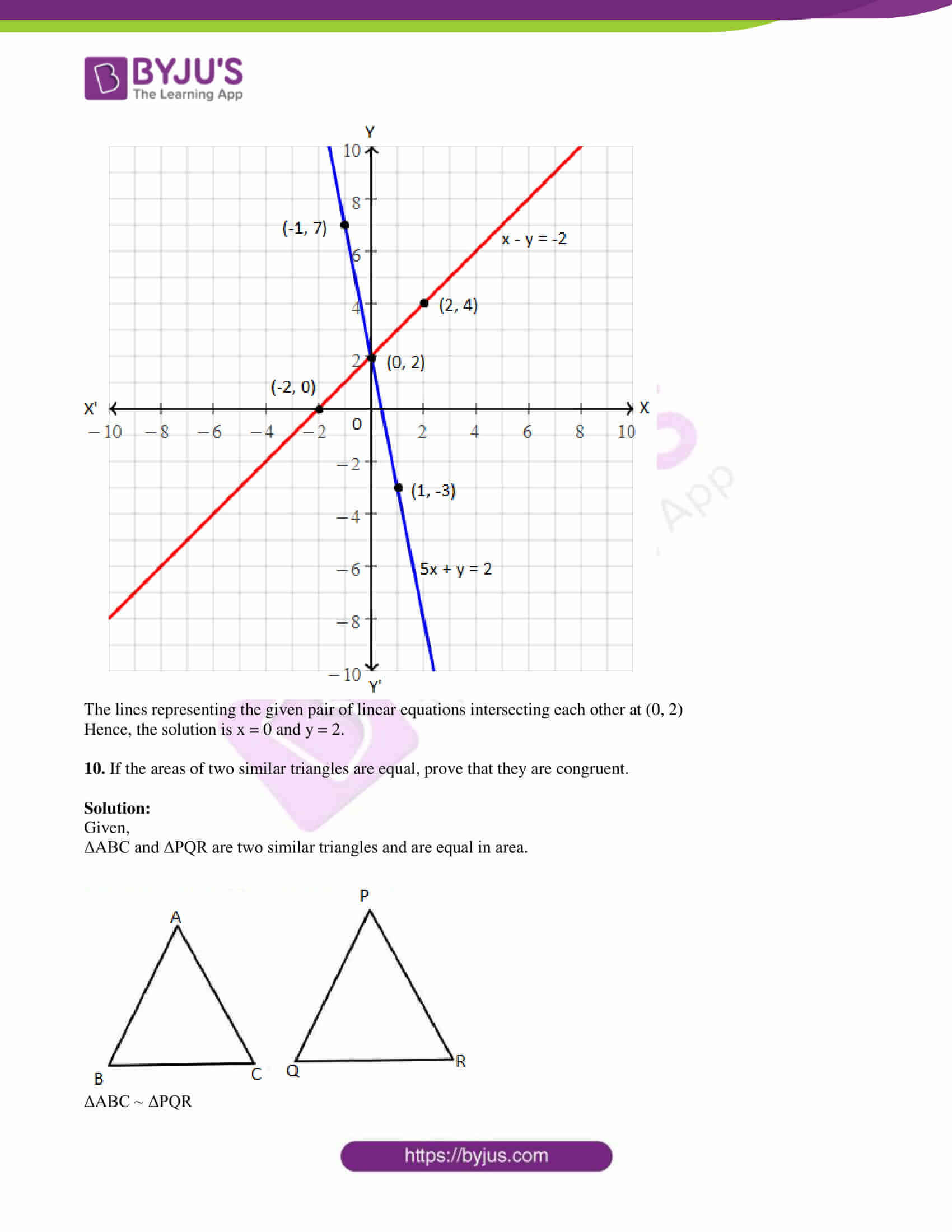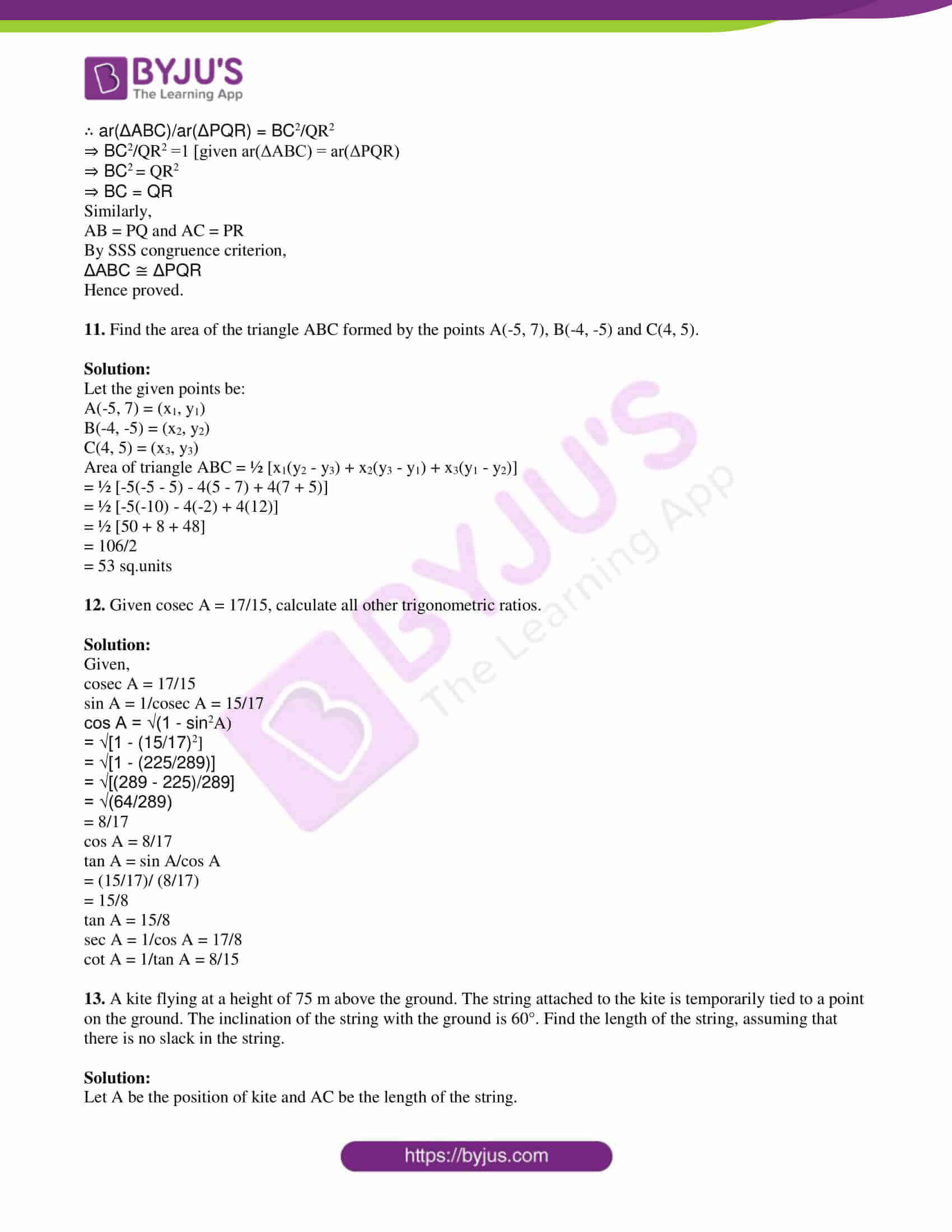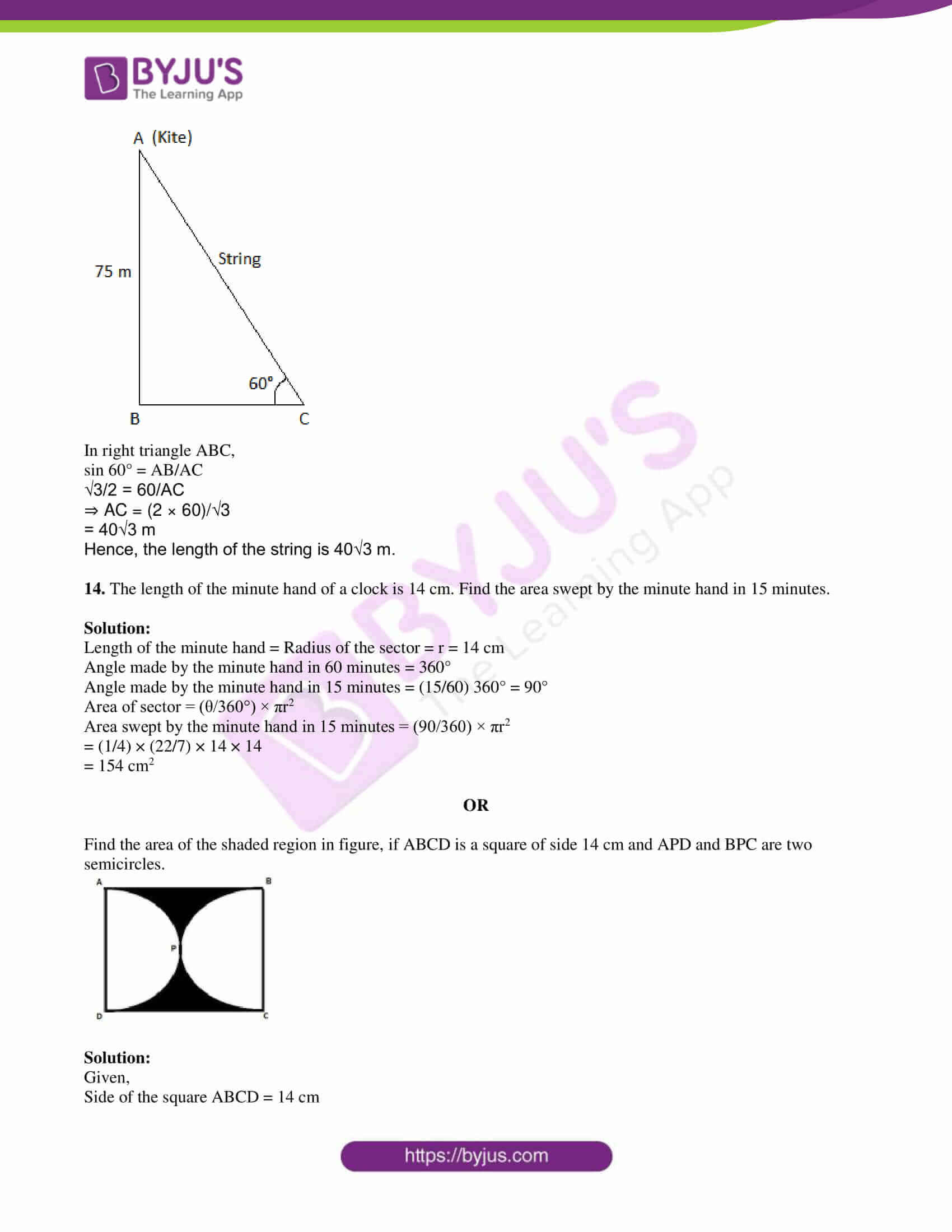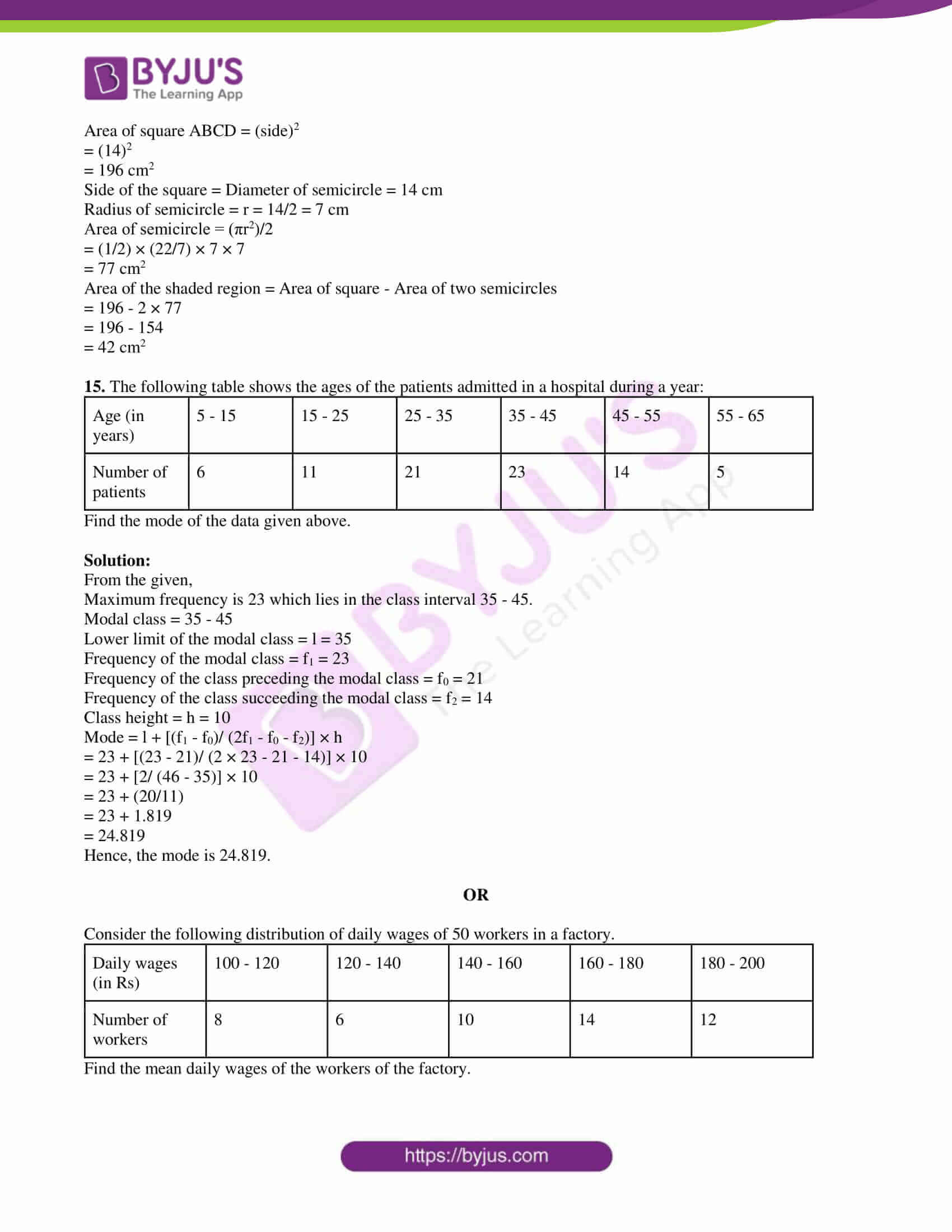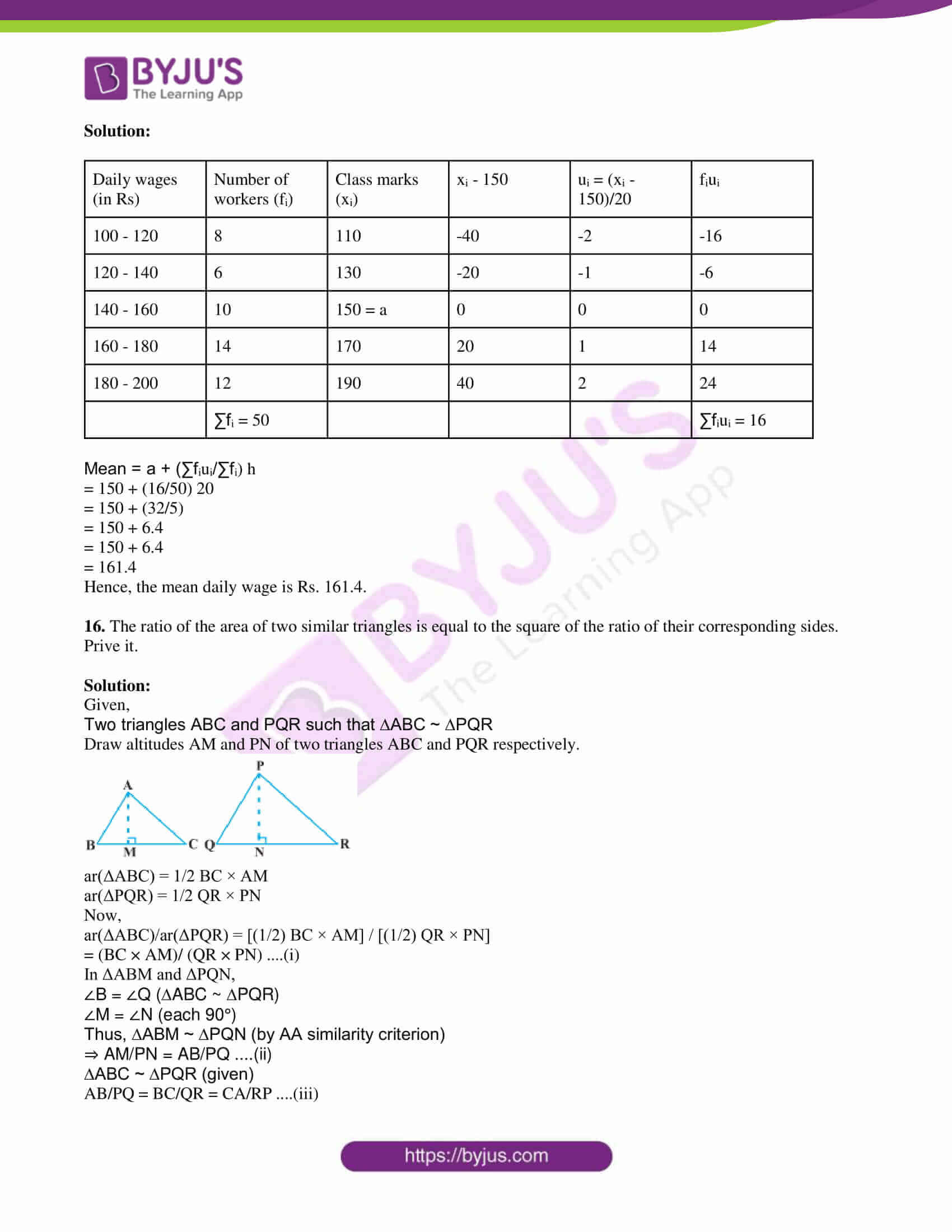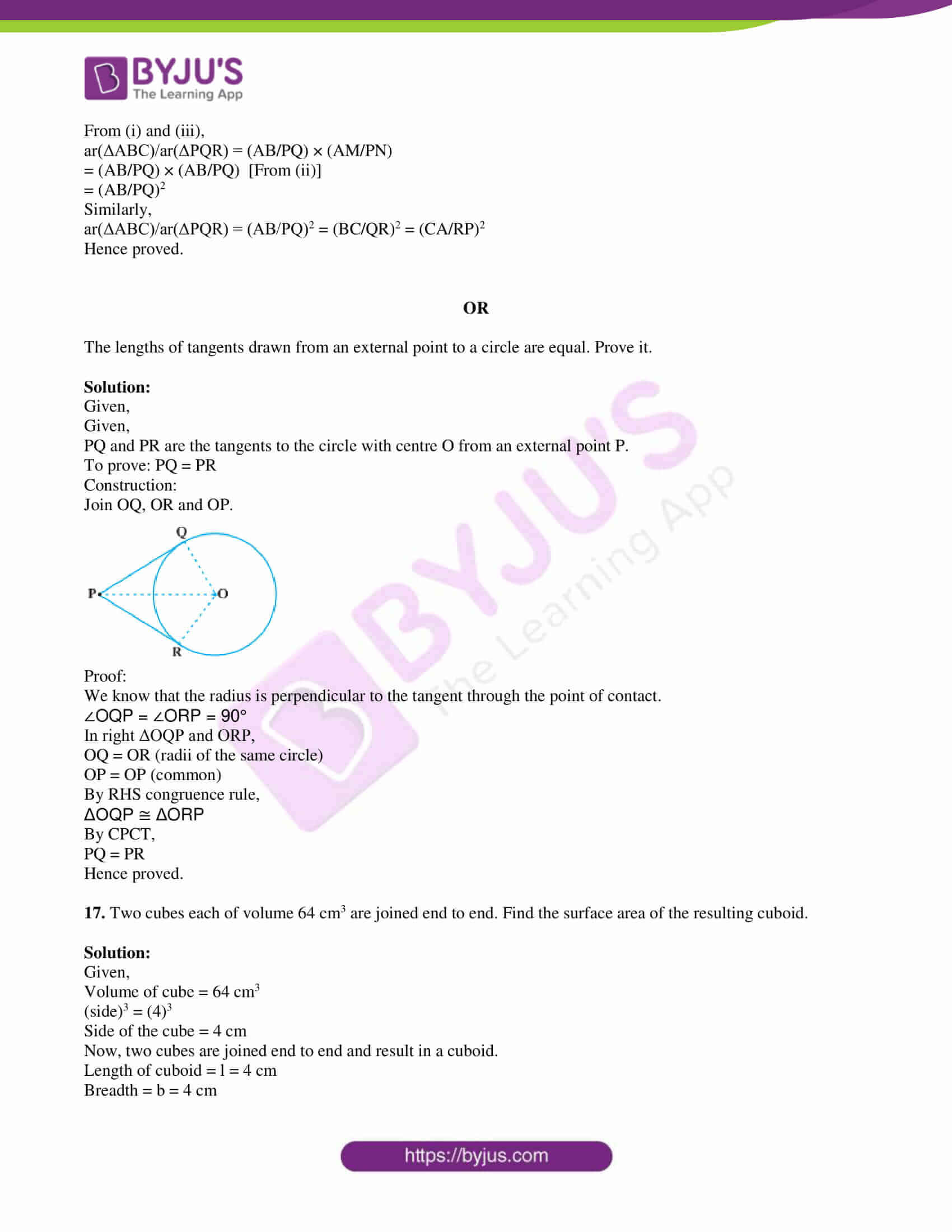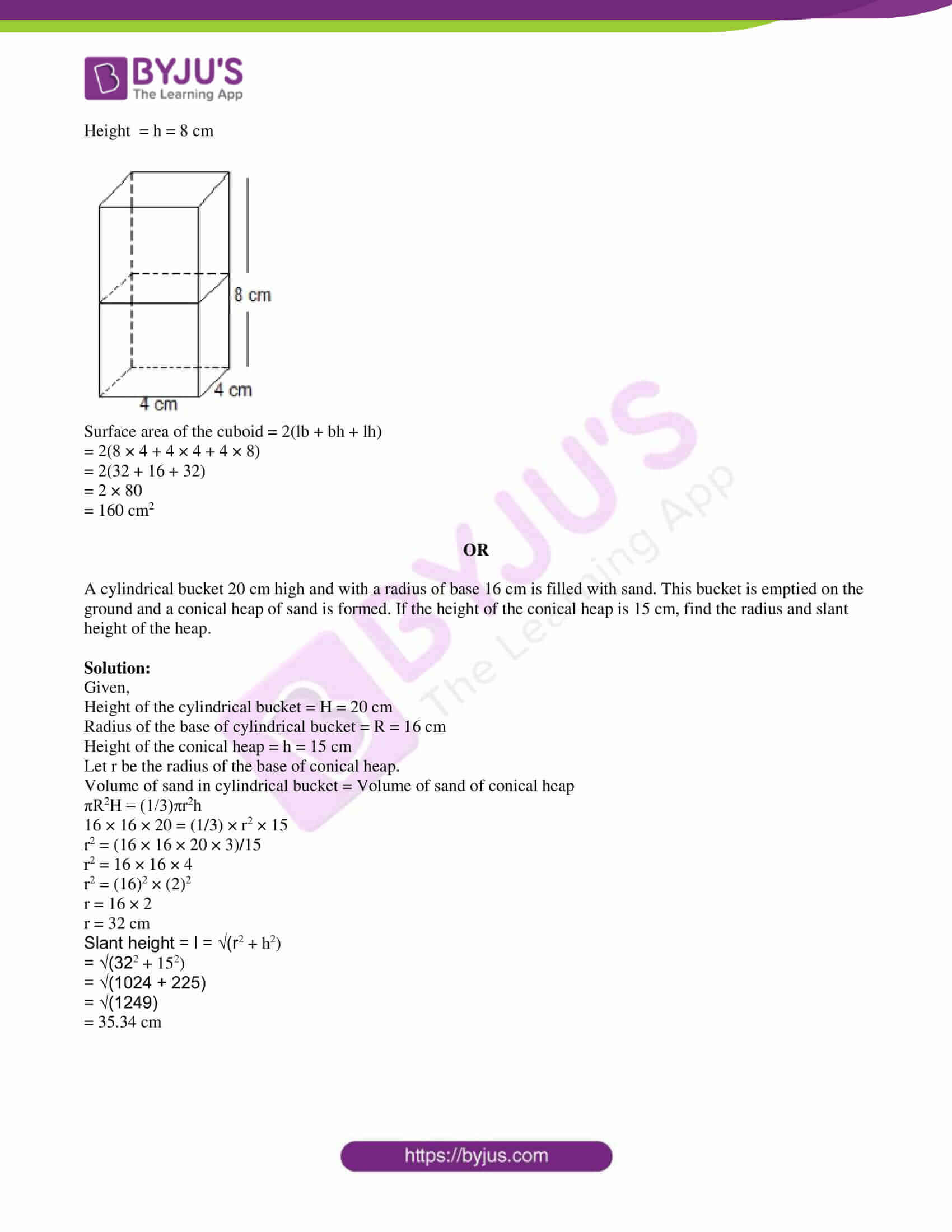Paper Code – 04/A

PART – A

1. (i) Find the value of x in the pair of linear equations 3x – y = 4 and 2x + y = 6.

Solution:

Given,

3x – y = 4….(i)

2x + y = 6….(ii)

3x – y + 2x + y = 4 + 6

5x = 10

x = 10/5

x = 2

(ii) Write the first four terms of AP, when first term a = 4 and common difference d = 3.

Solution:

Given,

First term = a = 4

Common difference = d = 3

Second term = a + d = 4 + 3 = 7

Third term = a + 2d = 4 + 2(3) = 4 + 6 = 10

Fourth term = a + 3d = 4 + 3(3) = 4 + 9 = 13

Hence, the first four terms of the AP are 4, 7, 10, 13.

(iii) Fill in the blanks (?):

If ΔABC ~ ΔDEF, then ar(ΔABC)/ar(ΔDEF) = AC2/?

Solution:

ar(ΔABC)/ar(ΔDEF) = AC2/DF2

(iv) Evaluate: cosec 59° – sec 31°

Solution:

cosec 59° – sec 31°

= cosec (90° – 31°) – sec 31°

= sec 31° – sec 31°

= 0

(v) The length, breadth and height of the hall is 14 m, 9 m, and 7 m respectively. Find the area of its floor.

Solution:

Given,

Length = l = 14 m

Breadth = b = 9 m

Height = h = 7 m

Area of the floor of hall = 2(l + b)h

= 2(14 + 9) × 7

= 2(23) × 7

= 46 × 7

= 322 m2

PART – B

2. Given that HCF(315, 657) = 9, find LCM(315, 657).

Solution:

Given,

HCF(315, 657) = 9

We know that,

LCM × HCF = Product of the two given numbers

LCM × 9 = 315 × 657

LCM = (315 × 657)/9

= 22995

Therefore, LCM(315, 657) = 22995

3. Find a quadratic polynomial, the sum and product of whose zeroes is -3 and 2, respectively.

Solution:

Given,

Sum of the zeroes = -3

Product of the zeroes = 2

Hence, the quadratic polynomial = x2 – (sum of the zeroes)x + (product of the zeroes)

= x2 – (-3)x + 2

= x2 + 3x + 2

4. Find the roots of quadratic equation 3x2 – 5x – 2 = 0, if they exist.

Solution:

Given,

3x2 – 5x – 2 = 0

Comparing with the standard form ax2 + bx + c = 0,

a = 3, b = -5 and c = -2

Discriminant = b2 – 4ac

= (-5)2 – 4(3)(-2)

= 25 + 24

= 49 > 0

Thus, the given quadratic equation has two real and distinct roots.

3x2 – 5x – 2 = 0

3x2 – 6x + x – 2 = 0

3x(x – 2) + 1(x – 2) = 0

(3x + 1)(x – 2) = 0

x = -⅓, x = 2

Therefore, the roots of the given quadratic equation are -⅓ and 2.

OR

Find two consecutive odd positive integers, the sum of whose squares is 202.

Solution:

Let x and (x + 2) be the two consecutive odd positive integers.

According to the given,

x2 + (x + 2)2 = 202

x2 + x2 + 4x + 4 = 202

2x2 + 4x + 4 = 202

2(x2 + 2x + 2) = 202

x2 + 2x + 2 – 101 = 0

x2 + 2x – 99 = 0

x2 + 11x – 9x – 99 = 0

x(x + 11) – 9(x + 11) = 0

(x – 9)(x + 11) = 0

x = 9, -11

The value of x cannot be negative.

Now, x + 2 = 9 + 2 = 11

Hence, the required numbers are 9 and 11.

5. Find the sum of the first 15 multiples of 8.

Solution:

The first 15 multiples of 8 are:

8, 16, 24, 32,…., 120

This is an AP with a = 8 and d = 8

n = 15

an = 120

Sum of first n terms

Sn = n/2(a + an)

S15 = (15/2) × (8 + 120)

= (15/2) × 128

= 15 × 64

= 960

Hence, the sum of the first 15 multiples of 8 is 960.

OR

Find the 33rd term of AP whose 11th term is 38 and the 16th term is 73.

Solution:

Let a be the first term and d be the common difference of an AP.

Given,

a11 = 38

a + 10d = 38….(i)

And

a16 = 73

a + 15d = 73….(ii)

Subtracting (i) from (ii),

a + 15d – (a + 10d) = 73 – 38

5d = 35

d = 35/5

d = 7

Substituting d = 7 in (i),

a + 10(7) = 38

a = 38 – 70

a = -32

a33 = a + 32d

= -32 + 32(7)

= -32 + 224

= 192

Hence, the 33rd term if the AP is 192.

6. In figure, if DE || AC and DF || AE. Prove that BF/FE = BE/EC.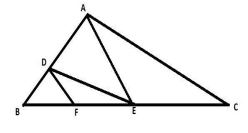Solution:

Given that in ΔABC,

DE || AC

By BPT,

∴BD/DA = BE/EC….(i)

In ΔABC,

DF || AE

By BPT,

∴BD/DA = BF/FE….(ii)

From (i) and (ii),

BE/EC = BF/FE

Hence proved.

OR

Let ΔABC ~ ΔDEF and their area be respectively 64 cm2 and 121 cm2. If EF = 13.2 cm, find BC.

Solution:

Given,

ΔABC ~ ΔDEF

ar(ΔABC) = 64 cm2

ar(ΔDEF) = 121 cm2

We know that the ratio of the areas of two similar triangles is equal to the squares of the ratio of their corresponding sides.

ar(ΔABC)/ ar(ΔDEF) = BC2/EF2

64/121 = BC2/(13.2)2

⇒ BC2 = (64 × 13.2 × 13.2)/121

= 92.16

⇒ BC = 9.6 cm

7. Draw a circle of radius 3 cm. From a point 7 cm away from its centre, construct the pair of tangents to the circle and measure their lengths.

Solution: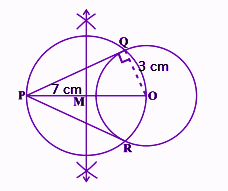PQ and PR are the required tangents to the circle.

PQ = PR = 6.325 cm (approx)

8. A child has a die whose six faces show the letters as given below.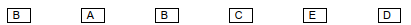The die is thrown once. What is the probability of getting (i) B (ii) E?

Solution:

Total number of outcomes = 6

i.e. {B, A, B, C, E, D}

(i) Number of B’s on die = 2

P(getting B) = 2/6 = 1/3

(ii) Number of E’s = 1

P(getting E) = 1/6

PART – C

9. The cost of 5 oranges and 3 apples is Rs. 35 and the cost of 2 oranges and 4 apples is Rs. 28. Find the cost of an orange and an apple.

Solution:

Let x be the cost (in Rs) of one orange and y be the cost (in Rs) of one apple.

According to the given,

5x + 3y = 35….(i)

2x + 4y = 28

2(x + 2y) = 28

x + 2y = 14

x = 14 – 2y….(ii)

Substituting (ii) in (i),

5(14 – 2y) + 3y = 35

70 – 10y + 3y = 35

7y = 70 – 35

7y = 35

y = 35/7 = 5

Substituting y = 5 in (ii),

x = 14 – 2(5) = 14 – 10 = 4

Hence, the cost of an orange is Rs. 4 and the cost of an apple is Rs. 5.

OR

Solve the pair of linear equations 5x + y = 2 and x – y = -2 graphically.

Solution:

Given,

5x + y = 2

x – y = -2

Consider the first equation:

5x + y = 2

y = -5x + 2

 x -1 0 1 y 7 2 -3

Now, consider another equation:

x – y = -2

y = x + 2

 x -2 0 2 y 0 2 4

Graph: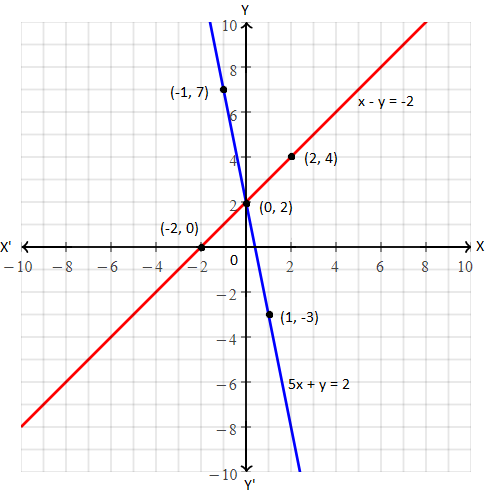The lines representing the given pair of linear equations intersecting each other at (0, 2)

Hence, the solution is x = 0 and y = 2.

10. If the areas of two similar triangles are equal, prove that they are congruent.

Solution:

Given,

ΔABC and ΔPQR are two similar triangles and are equal in area.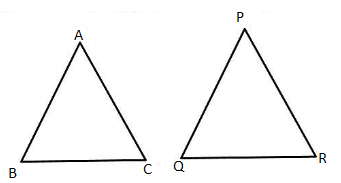ΔABC ~ ΔPQR

∴ ar(ΔABC)/ar(ΔPQR) = BC2/QR2

⇒ BC2/QR2 =1 [given ar(ΔABC) = ar(ΔPQR)

⇒ BC2 = QR2

⇒ BC = QR

Similarly,

AB = PQ and AC = PR

By SSS congruence criterion,

ΔABC ≅ ΔPQR

Hence proved.

11. Find the area of the triangle ABC formed by the points A(-5, 7), B(-4, -5) and C(4, 5).

Solution:

Let the given points be:

A(-5, 7) = (x1, y1)

B(-4, -5) = (x2, y2)

C(4, 5) = (x3, y3)

Area of triangle ABC = ½ [x1(y2 – y3) + x2(y3 – y1) + x3(y1 – y2)]

= ½ [-5(-5 – 5) – 4(5 – 7) + 4(7 + 5)]

= ½ [-5(-10) – 4(-2) + 4(12)]

= ½ [50 + 8 + 48]

= 106/2

= 53 sq.units

12. Given cosec A = 17/15, calculate all other trigonometric ratios.

Solution:

Given,

cosec A = 17/15

sin A = 1/cosec A = 15/17

cos A = √(1 – sin2A)

= √[1 – (15/17)2]

= √[1 – (225/289)]

= √[(289 – 225)/289]

= √(64/289)

= 8/17

cos A = 8/17

tan A = sin A/cos A

= (15/17)/ (8/17)

= 15/8

tan A = 15/8

sec A = 1/cos A = 17/8

cot A = 1/tan A = 8/15

13. A kite flying at a height of 75 m above the ground. The string attached to the kite is temporarily tied to a point on the ground. The inclination of the string with the ground is 60°. Find the length of the string, assuming that there is no slack in the string.

Solution:

Let A be the position of kite and AC be the length of the string.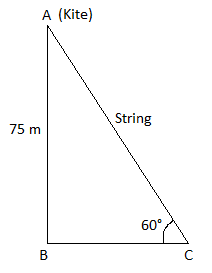In right triangle ABC,

sin 60° = AB/AC

√3/2 = 60/AC

⇒ AC = (2 × 60)/√3

= 40√3 m

Hence, the length of the string is 40√3 m.

14. The length of the minute hand of a clock is 14 cm. Find the area swept by the minute hand in 15 minutes.

Solution:

Length of the minute hand = Radius of the sector = r = 14 cm

Angle made by the minute hand in 60 minutes = 360°

Angle made by the minute hand in 15 minutes = (15/60) 360° = 90°

Area of sector = (θ/360°) × πr2

Area swept by the minute hand in 15 minutes = (90/360) × πr2

= (1/4) × (22/7) × 14 × 14

= 154 cm2

OR

Find the area of the shaded region in figure, if ABCD is a square of side 14 cm and APD and BPC are two semicircles.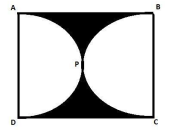Solution:

Given,

Side of the square ABCD = 14 cm

Area of square ABCD = (side)2

= (14)2

= 196 cm2

Side of the square = Diameter of semicircle = 14 cm

Radius of semicircle = r = 14/2 = 7 cm

Area of semicircle = (πr2)/2

= (1/2) × (22/7) × 7 × 7

= 77 cm2

Area of the shaded region = Area of square – Area of two semicircles

= 196 – 2 × 77

= 196 – 154

= 42 cm2

15. The following table shows the ages of the patients admitted in a hospital during a year:

 Age (in years) 5 – 15 15 – 25 25 – 35 35 – 45 45 – 55 55 – 65 Number of patients 6 11 21 23 14 5

Find the mode of the data given above.

Solution:

From the given,

Maximum frequency is 23 which lies in the class interval 35 – 45.

Modal class = 35 – 45

Lower limit of the modal class = l = 35

Frequency of the modal class = f1 = 23

Frequency of the class preceding the modal class = f0 = 21

Frequency of the class succeeding the modal class = f2 = 14

Class height = h = 10

Mode = l + [(f1 – f0)/ (2f1 – f0 – f2)] × h

= 23 + [(23 – 21)/ (2 × 23 – 21 – 14)] × 10

= 23 + [2/ (46 – 35)] × 10

= 23 + (20/11)

= 23 + 1.819

= 24.819

Hence, the mode is 24.819.

OR

Consider the following distribution of daily wages of 50 workers in a factory.

 Daily wages (in Rs) 100 – 120 120 – 140 140 – 160 160 – 180 180 – 200 Number of workers 8 6 10 14 12

Find the mean daily wages of the workers of the factory.

Solution:

 Daily wages (in Rs) Number of workers (fi) Class marks (xi) xi – 150 ui = (xi – 150)/20 fiui 100 – 120 8 110 -40 -2 -16 120 – 140 6 130 -20 -1 -6 140 – 160 10 150 = a 0 0 0 160 – 180 14 170 20 1 14 180 – 200 12 190 40 2 24 ∑fi = 50 ∑fiui = 16

Mean = a + (∑fiui/∑fi) h

= 150 + (16/50) 20

= 150 + (32/5)

= 150 + 6.4

= 150 + 6.4

= 161.4

Hence, the mean daily wage is Rs. 161.4.

16. The ratio of the area of two similar triangles is equal to the square of the ratio of their corresponding sides. Prive it.

Solution:

Given,

Two triangles ABC and PQR such that ∆ABC ~ ∆PQR

Draw altitudes AM and PN of two triangles ABC and PQR respectively.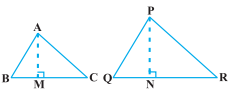ar(ΔABC) = 1/2 BC × AM

ar(ΔPQR) = 1/2 QR × PN

Now,

ar(ΔABC)/ar(ΔPQR) = [(1/2) BC × AM] / [(1/2) QR × PN]

= (BC × AM)/ (QR × PN) ….(i)

In ΔABM and ΔPQN,

∠B = ∠Q (∆ABC ~ ∆PQR)

∠M = ∠N (each 90°)

Thus, ∆ABM ~ ∆PQN (by AA similarity criterion)

⇒ AM/PN = AB/PQ ….(ii)

∆ABC ~ ∆PQR (given)

AB/PQ = BC/QR = CA/RP ….(iii)

From (i) and (iii),

ar(ΔABC)/ar(ΔPQR) = (AB/PQ) × (AM/PN)

= (AB/PQ) × (AB/PQ) [From (ii)]

= (AB/PQ)2

Similarly,

ar(ΔABC)/ar(ΔPQR) = (AB/PQ)2 = (BC/QR)2 = (CA/RP)2

Hence proved.

OR

The lengths of tangents drawn from an external point to a circle are equal. Prove it.

Solution:

Given,

Given,

PQ and PR are the tangents to the circle with centre O from an external point P.

To prove: PQ = PR

Construction:

Join OQ, OR and OP.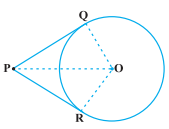Proof:

We know that the radius is perpendicular to the tangent through the point of contact.

∠OQP = ∠ORP = 90°

In right ΔOQP and ORP,

OQ = OR (radii of the same circle)

OP = OP (common)

By RHS congruence rule,

ΔOQP ≅ ΔORP

By CPCT,

PQ = PR

Hence proved.

17. Two cubes each of volume 64 cm3 are joined end to end. Find the surface area of the resulting cuboid.

Solution:

Given,

Volume of cube = 64 cm3

(side)3 = (4)3

Side of the cube = 4 cm

Now, two cubes are joined end to end and result in a cuboid.

Length of cuboid = l = 4 cm

Breadth = b = 4 cm

Height = h = 8 cm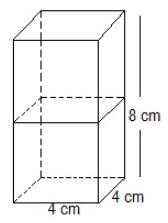Surface area of the cuboid = 2(lb + bh + lh)

= 2(8 × 4 + 4 × 4 + 4 × 8)

= 2(32 + 16 + 32)

= 2 × 80

= 160 cm2

OR

A cylindrical bucket 20 cm high and with a radius of base 16 cm is filled with sand. This bucket is emptied on the ground and a conical heap of sand is formed. If the height of the conical heap is 15 cm, find the radius and slant height of the heap.

Solution:

Given,

Height of the cylindrical bucket = H = 20 cm

Radius of the base of cylindrical bucket = R = 16 cm

Height of the conical heap = h = 15 cm

Let r be the radius of the base of conical heap.

Volume of sand in cylindrical bucket = Volume of sand of conical heap

πR2H = (1/3)πr2h

16 × 16 × 20 = (1/3) × r2 × 15

r2 = (16 × 16 × 20 × 3)/15

r2 = 16 × 16 × 4

r2 = (16)2 × (2)2

r = 16 × 2

r = 32 cm

Slant height = l = √(r2 + h2)

= √(322 + 152)

= √(1024 + 225)

= √(1249)

= 35.34 cm